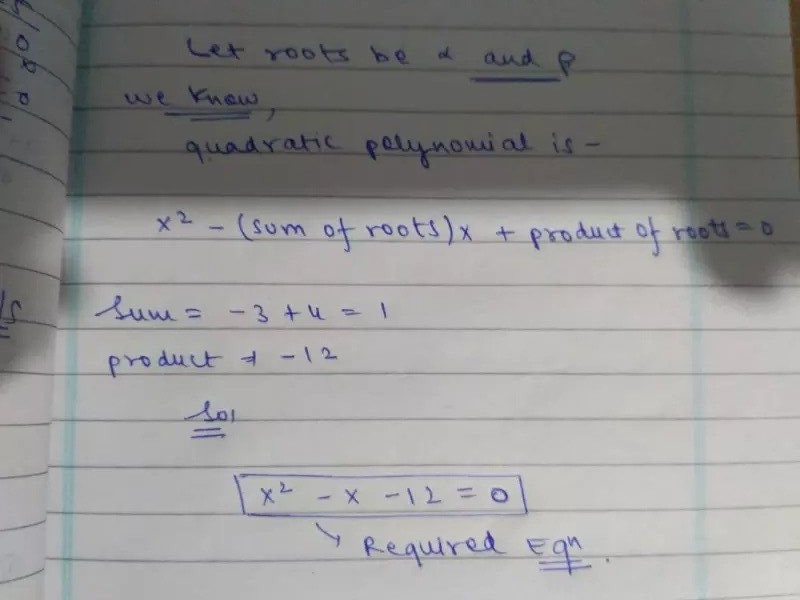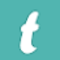Find the quadratic polynomial whose zeroes are (-3) and 4.## Text Version of the answer is

Let roots be x and p

We know

X 2 − (sum of roots) x + product of roots = 0

Sum = −3 + 4 = 1

Product = −12

So

x 2 − x − 12 = 0

Required E9n